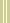January 20, 2000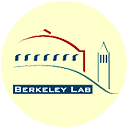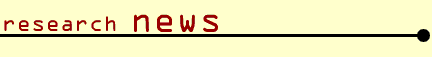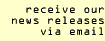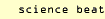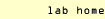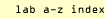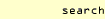BERKELEY, CA — Among the top ten "Algorithms of the Century" announced in the January/February, 2000, issue of Computing in Science and Engineering magazine is the integer-relation algorithm dubbed PSLQ, discovered by mathematician and sculptor Helaman Ferguson of Maryland's Center for Computing Sciences, and implemented in practical computer software by David Bailey, chief technologist of the National Energy Research Scientific Computing Center (NERSC) at the Department of Energy's Lawrence Berkeley National Laboratory.##### A SIMPLE FORMULA DISCOVERED WITH PSLQ MAKES IT POSSIBLE TO CALCULATE THE NTH BINARY DIGIT OF PI WITHOUT COMPUTING ANY OF THE FIRST N-1 DIGITS, AND DO THE COMPUTATION WITH VERY LITTLE COMPUTING POWER.

PSLQ has unearthed many surprising relations in mathematics and physics, although its most startling result may well be a simple formula for calculating any binary digit of pi without calculating the digits preceding it. Before PSLQ, mathematicians had not thought that such a digit-extraction algorithm for pi was possible.

Using the remarkably simple formula, even a personal computer can calculate pi's millionth binary digit in about 60 seconds. Most applications of PSLQ, however, require much more computing power and must employ much greater numerical precision than the standard 16-digit, 64-bit, floating-point arithmetic available on most computers.

"Sixteen-digit arithmetic is sufficient for almost all scientific applications," says Bailey, "but a few crazy people need more. I'm one."

Bailey has developed software that translates ordinary FORTRAN programs into programs capable of arbitrary precision -- calculations accurate to tens, hundreds, or even many thousands of digits. Real physical problems that require this kind of "multiprecision" include simulating the dynamics of vortices, in which 80- to 100-digit arithmetic is needed for extremely high accuracy in each of many sequential calculations, to avoid meaningless results at the end.

As a tool of experimental mathematics, PSLQ's purpose is to discover new mathematical relations among numbers -- for example, constants that occur in various groups of mathematical formulas. Very high precision arithmetic is needed by PSLQ, or else nonsense results are obtained.

"Integer-relation detection is an old problem, first studied by Euclid about 300 BC," Bailey says. "He came up with an algorithm for finding the relation, if one exists, between any two numbers. Later many famous mathematicians -- Euler, Jacobi, Poincaré, Minkowski, and others -- tried to generalize Euclid's algorithm to any 'n' numbers, without success. Ferguson finally found a general algorithm in 1977, and recently found a much more efficient algorithm, named PSLQ. But this new method wasn't practical until it could be implemented with multiprecision software on high-performance computer systems."

Bailey calls PSLQ "a computer scheme to discover new mathematical results." In a short time it has found polylogarithmic formulas in algebraic number theory, identified a class of multiple-sum constants, uncovered relations in the renormalization procedures of quantum field theory symbolized by Feynman diagrams, and found a formula for calculating any digit of pi.

Some of these results have profound implications. The pi formula raises questions about the long-held but never proved assumption that pi's digits are random. The Feynman-diagram results suggest unsuspected relationships among formulas associated with fundamental particles.

PSLQ, an efficient, numerically stable algorithm guaranteed to find relations among integers in a limited number of iterations, is a shining example of modern computer technology applied to the discovery of new facts of mathematics and physics -- truly an algorithm of the century. It's also a harbinger of the future: experimental mathematics using computers will become increasingly important in the coming century.

The Berkeley Lab is a U.S. Department of Energy national laboratory located in Berkeley, California. It conducts unclassified scientific research and is managed by the University of California.